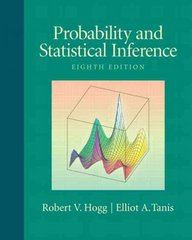Angelo State University Textbooks > Probability and Statistical Inference

# Probability and Statistical Inference 8th editionISBN:0321584759

ISBN13:9780321584755

Publisher:Pearson

Authors:Robert V. Hogg, Elliot Tanis

Renting Options

Own it? Sell it back

## Probability and Statistical Inference Description

Probability and Statistical Inference 8 Edition BOOK DESCRIPTION: Written by two leading statisticians, this applied introduction to the mathematics of probability and statistics emphasizes the existence of variation in almost every process, and how the study of probability and statistics helps us understand this variation. Designed for students with a background in calculus, this book continues to reinforce basic mathematical concepts with numerous real-world examples and applications to illustrate the relevance of key concepts.   NEW TO THIS EDITION: The included CD-ROM contains all of the data sets in a variety of formats for use with most statistical software packages. This disc also includes several applications of Minitab® and Maple™. Historical vignettes at the end of each chapter outline the origin of the greatest accomplishments in the field of statistics, adding enrichment to the course.  Content updates The first five chapters have been reorganized to cover a standard probability course with more real examples and exercises. These chapters are important for students wishing to pass the first actuarial exam, and cover the necessary material needed for students taking this course at the junior level. Chapters 6 and 7 on estimation and tests of statistical hypotheses tie together confidence intervals and tests, including one-sided ones. There are separate chapters on nonparametric methods, Bayesian methods, and Quality Improvement. Chapters 4 and 5 include a strong discussion on conditional distributions and functions of random variables, including Jacobians of transformations and the moment-generating technique. Approximations of distributions like the binomial and the Poisson with the normal can be found using the central limit theorem. Chapter 8 (Nonparametric Methods) includes most of the standards tests such as those by Wilcoxon and also the use of order statistics in some distribution-free inferences. Chapter 9 (Bayesian Methods) explains the use of the "Dutch book" to prove certain probability theorems. Chapter 11 (Quality Improvement) stresses how important W. Edwards Deming's ideas are in understanding variation and how they apply to everyday life. TABLE OF CONTENTS:  Preface Prologue   1. Probability 1.1 Basic Concepts 1.2 Properties of Probability 1.3 Methods of Enumeration 1.4 Conditional Probability 1.5 Independent Events 1.6 Bayes's Theorem   2. Discrete Distributions 2.1 Random Variables of the Discrete Type 2.2 Mathematical Expectation 2.3 The Mean, Variance, and Standard Deviation 2.4 Bernoulli Trials and the Binomial Distribution 2.5 The Moment-Generating Function 2.6 The Poisson Distribution   3. Continuous Distributions 3.1 Continuous-Type Data 3.2 Exploratory Data Analysis 3.3 Random Variables of the Continuous Type 3.4 The Uniform and Exponential Distributions 3.5 The Gamma and Chi-Square Distributions 3.6 The Normal Distribution 3.7 Additional Models   4. Bivariate Distributions 4.1 Distributions of Two Random Variables 4.2 The Correlation Coefficient 4.3 Conditional Distributions 4.4 The Bivariate Normal Distribution   5. Distributions of Functions of Random Variables 5.1 Functions of One Random Variable 5.2 Transformations of Two Random Variables 5.3 Several Independent Random Variables 5.4 The Moment-Generating Function Technique 5.5 Random Functions Associated with Normal Distributions 5.6 The Central Limit Theorem 5.7 Approximations for Discrete Distributions   6. Estimation 6.1 Point Estimation 6.2 Confidence Intervals for Means 6.3 Confidence Intervals for Difference of Two Means 6.4 Confidence Intervals for Variances 6.5 Confidence Intervals for Proportions 6.6 Sample Size. 6.7 A Simple Regression Problem 6.8 More Regression   7. Tests of Statistical Hypotheses 7.1 Tests about Proportions 7.2

## Employers & Housing Providers

Employers can list job opportunities for students

Housing Providers can list available housing

## You just missed it! This listing has been filled.

Post your own housing listing on Uloop and have students reach out to you!